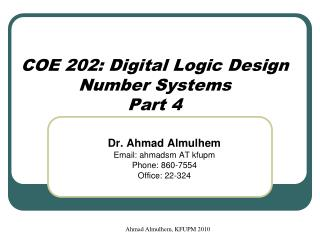Download PresentationCOE 202: Digital Logic Design Number Systems Part 4

# COE 202: Digital Logic Design Number Systems Part 4 - PowerPoint PPT PresentationDownload Presentation## COE 202: Digital Logic Design Number Systems Part 4

- - - - - - - - - - - - - - - - - - - - - - - - - - - E N D - - - - - - - - - - - - - - - - - - - - - - - - - - -
##### Presentation Transcript

1. Ahmad Almulhem, KFUPM 2010 Dr. Ahmad Almulhem Email: ahmadsm AT kfupm Phone: 860-7554 Office: 22-324 COE 202: Digital Logic DesignNumber SystemsPart 4

2. Ahmad Almulhem, KFUPM 2010 Objectives • Binary codes • Binary coded decimal (BCD) • Other Decimal Codes • Gray Code • ASCII Code • Error Detecting Code

3. Ahmad Almulhem, KFUPM 2010 Binary Codes • A n-bit binary code is a binary string of 0s and 1s of size n. • It can represent 2n different elements. • 4 elements can coded using 2 bits • 8 elements can be coded using 3 bits • Given the number of elements to be coded, there is a minimum number of bits, but no maximum !

4. Ahmad Almulhem, KFUPM 2010 Binary Coded Decimal (BCD) • Human communicating with computers • Humans understand decimal • Computers understands binary • Solution: Convert Decimal-Binary-Decimal • Need to store decimal numbers as binary codes

5. Ahmad Almulhem, KFUPM 2010 Binary Coded Decimal (BCD) • BCD Code uses 4 bits to represent the 10 decimal digits {0 to 9} • 6 BCD codes unused • The weights of the individual positions of the bits of a BCD code are: 23=8, 22=4, 21=2, 20=1

7. Ahmad Almulhem, KFUPM 2010 Other Decimal Codes • 4 bits = 16 different codes • Only 10 needed to represent the 10 decimal digits. • Many possible codes! • 2421 and excess-3 are self-complementing (9’s complement can be obtained by inverting bits) src: Mano’s book

8. Ahmad Almulhem, KFUPM 2010 Gray Code • Gray code represents decimal numbers 0 to 15 using 16 4-bit codes • Gray codes of two adjacent decimal numbers differ by only one bit • Example: • (5)10 = 0111 • (6)10 = 0101 • (7)10 = 0100

9. Ahmad Almulhem, KFUPM 2010 ASCII Character Code • ASCII an abbreviation of “American Standard Code for Information Interchange” • A 7-bit code (128 characters) • 94 printable, 34 non-printable (control) • 2x26 English letters (A,…Z, a,…z) • 10 decimal digits (0,1,…9) • 32 Special Characters such as %, *, \$, … etc. • Usually stored as a byte (8 bits) • The extra bit is used for other purposes

10. Ahmad Almulhem, KFUPM 2010 ASCII Character Code

11. Ahmad Almulhem, KFUPM 2010 ASCII Character Code capital vs small A difference of (20)16 = 3210

12. Ahmad Almulhem, KFUPM 2010 Error Detecting Code • In data communication, errors may happen • One code change into another code • How to detect errors? • Add an extra bit called a parity bit such that • Number of 1’s is even (even parity) or odd (odd parity)

13. Ahmad Almulhem, KFUPM 2010 Error Detecting Code ASCII A = ASCII T =

14. Ahmad Almulhem, KFUPM 2010 Conclusions • Bits are bits • Modern digital devices represent everything as collections of bits • A computer is one such digital device • You can encode anything with sufficient 1’s and 0’s • Binary codes (BCD, gray code) • Text (ASCII) • Sound (.wav, .mp3, ...) • Pictures (.jpg, .gif, .tiff)